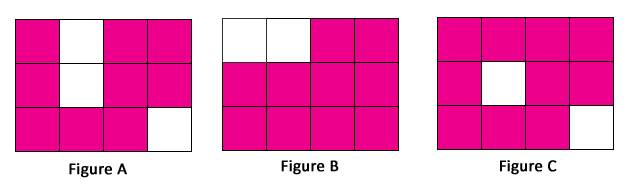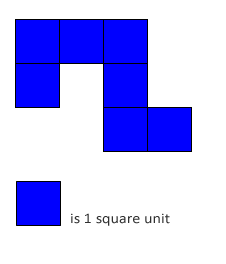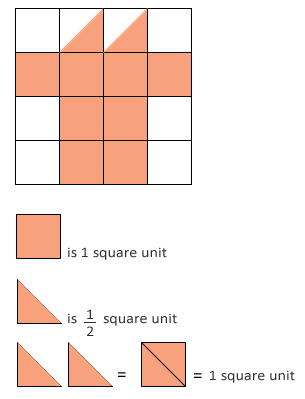## Area in Square Units

Practice Unlimited Questions

#### What is an area?

Area is the size of a surface or the amount of surface covered.

#### 1. Falaq makes the following figures using identical square tiles. What is the area of each figure? Which two figures have the same area?Figure A is made up of 9 square tiles. Its area is 9 tiles.
Figure B is made up of 10 square tiles. Its area is 10 tiles.
Figure C is made up of 10 square tiles. Its area is 10 tiles.

Figure B and Figure C are made up of 10 tiles each.
They have the same area.
Two different shapes can have the same area if they use the same number of tiles.

#### 2. What is the area of the shaded figure below?